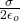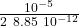## A cell membrane consists of an inner and outer wall separated by a distance of approximately 10nm10nm. Assume that the walls act like a para

Question

A cell membrane consists of an inner and outer wall separated by a distance of approximately 10nm10nm. Assume that the walls act like a parallel plate capacitor, each with a charge density of 10−5C/m2, and the outer wall is positively charged. Although unrealistic, assume that the space between cell walls is filled with air.
1. What is the magnitude of the electric field between the membranes?
A. 1×10^−15N/C
B. 5×10^−5N/C
C. 1×10^6N/C
D. 9×10^−2N/C
2. What is the magnitude of the force on a Ca++ ion between the cell walls?
A. 4×10^−13N
B. 4×10^−12N
C. 2×10^−12N
D. 2×10^−11N
3. What is the potential difference between the cell walls?
A. 1×10^7V
B. 1×10^−2V
C. 6×10^−3V
D. 10V
4. What is the direction of the electric field between the walls?
A. Toward the outer wall.
B. Parallel to the walls.
C. Toward the inner wall.
D. There is no electric field.
5. If released from the inner wall, what would be the kinetic energy of a 3fC charge at the outer wall? 1fC=10^−15C.
A. 3×10^−14J
B. 3×10^−17J
C. 3×10^−8J
D. 3×10^−2J

in progress 0
2 weeks 2021-08-29T19:08:22+00:00 1 Answers 1 views 0

the correct answue are  B, A, C, C, B

Explanation:

1) The electric field is requested, let’s approximate the membrane by a parallel plate with surface charge density

E =E =E = 5.65 10⁵ N / C

2) A calcium ion has two positive charges, so the force applied by each side of the membrane (plate)

F = q E

F = 2  1.6 10⁻¹⁹  5.65 10⁵

F = 1.8 10⁻¹³ N

the total force is the sum of the force of each membrane and the two forces go to the same side

F = total = 2 F

F_total = 3.6 10⁻¹³ N

3) the field and the electric potential are related

ΔV = – E s

ΔV = – 5.65 10⁵  10 10⁻⁹

ΔV = – 5.65 10⁻³ V

4) In the exercise they indicate that the outer wall has a positive charge, therefore, as they indicate that we approximate the system to a capacitor, the inner wall must be negatively charged.

The electric field goes from the positive to the negative charge, which is why it goes from the outer wall to the inner wall

5) For this part we use conservation of energy

starting point. On the inside wall, brown

Em₀ = U = qV

final point. On the outside

Em_f = K

energy is conserved

Em₀ = Em_f

q V = K

K = 3 10⁻¹⁵  5.65 10⁻³

K = 1.7 10⁻¹⁷ J